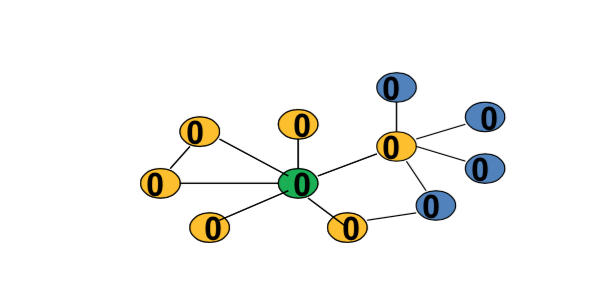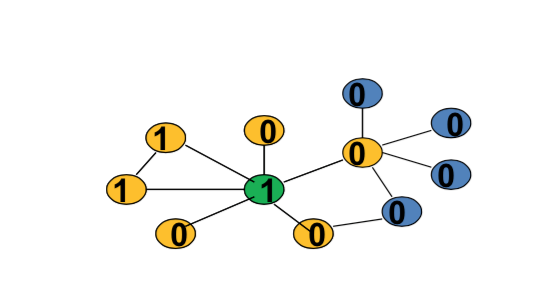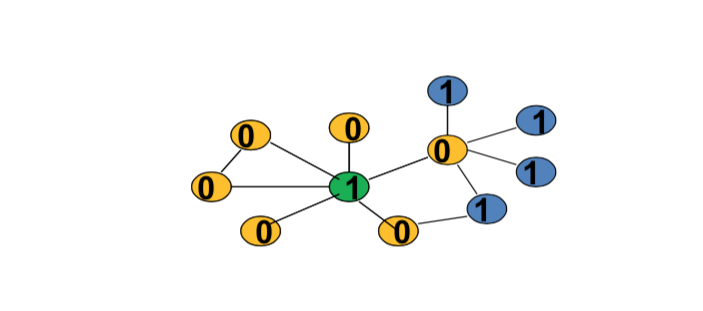# Outline¶

• Part 1: Background and Fundamentals

• Definitions and Characteristics of Networks(1,2)
• Empirical Background(3)
• Part 2: Network Formation

• Random Network Models (4,5)
• Strategic Network Models (6,11)
• Part 3: Networks and Behavior

• Diffusion and Learning (7,8)
• Games on Networks (9)

# Games on Networks¶

• Decisions to be made

• not just diffusion
• not just updating beliefs
• Complementarities...

• It's that people care about what other individuals are doing, so there's complementarities
• "Strategic" Interplay

• Inter-dependencies

I want to only buy a certain program if other people are using that same program. So the way in which I write articles depends on what my co-authors are doing, or I want to learn a certain language only if other people are also speaking that language. So there's going to be inter-dependencies between what individuals do. And there could also be situations where I can free ride. So if somebody else buys a new book, I can borrow it from them and maybe then I don't buy it myself. So who I know that's actually bought a book, maybe that affects whether I buy the book, both positively and negatively. So there's strategic inter-dependencies. And you know, the idea of games, people think of games - you know, we're not talking about Monopoly or chess, checkers etc. We're thinking about a situation where there's interactions. And what a given individual is going to do depends on what other individuals are doing, so there is some game aspect to it in that sense. But we're using game theory as a tool to try and understand exactly how behavior relates to network structure.

# Games on Networks - Outline¶

• Basic Definitions

• Examples

• Strategic Complements / Substitutes

• Equilibrium existence and structure

• Equilibrium response to network structure

# Games on Networks¶

• Players on a network - explicitly modeled...

• Care about actions of neighbors

• Early literature: How complex is the computation of equilibrium in worst case games?

• Second branch: what can we say about behavior and how it relates to network structure

# Start with a Canonical Special Case¶

• Each player chooses action $x_i$ in {0,1}

• e.g. buy the book or don't buy the book, or learn a language or don't learn a language
• payoff will depend on

• how many neighbors choose each action
• how many neighbors a players has

# Definitions¶

• Each player chooses action $x_i$ in {0,1}

• Consider cases where i's payoff is $$u_{d_i}(x_i, m_{N_i})$$

• depends only on $d_i(g)$ and $m_{N_i(g)}$ - the number of neighbors of i choosing 1
• i.e. only care about the number of friends taking the action.

# Example: Simple Complement¶

• agent i is willing to choose 1 if and only if at least t neightbors do

• payoff action 0: $$u_{d_i}(0, m_{N_i})=0$$

• Payoff action 1: $$u_{d_i}(1, m_{N_i}) = -t + m_{N_i}$$

• t: is threshold

# Example¶

• An agent is willing to take action 1 if and only if at least two neighbors do# Example: Best Shot¶

• agent i is willing to choose 1 if and only if no neightbors do
• let's think of the example where if somebody else one of my friends buys the book, I don't buy the book because now I can borrow it from them, so I'm willing to buy the book if and only if none of my neighbors do.
• payoff action 0:

• $u_{d_i}(0, m_{N_i})= 1$ if $m_{N_i} > 0$. If I don't buy the book(action 0), my payoff is 1 if some of my neighbors buys the book($m_{N_i} > 0$), I borror it from them
• $u_{d_i}(0, m_{N_i})= 0$ if $m_{N_i} = 0$. If none of my neighbors bought the book($m_{N_i} = 0$), I can't borrow it, I get a pay off of zero.
• Payoff action 1:

• $u_{d_i}(1, m_{N_i}) = 1 - c$. Stead, I buy it myself(action 1), then the payoff is 1-c
• c: cost of the book.

My optimal payoff would be I'd love to have one of my friends buy it($1 > 1-c >0$), me not buy it and borrow it from them. My worst payoff is nobody buys it and I don't buy it.

# Another Example: Best Shot Public Goods¶

• An agent is willing to take action 1 if and only if no neighbors doIn [ ]: